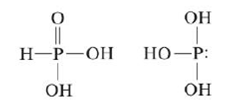# Many structures of phosphorus-containing compounds are drawn with some P9O bonds. These bonds are not the typical π bonds we’ve considered, which involve the overlap of two p orbitals. Instead, they result from the overlap of a d orbital on the phosphorus atom with a p orbital on oxygen. This type of π bonding is sometimes used as an explanation for why H 3 PO 3 has the first structure below rather than the second: Draw a picture showing how a d orbital and a p orbital overlap to form a π bond.### Chemistry: An Atoms First Approach

2nd Edition
Steven S. Zumdahl + 1 other
Publisher: Cengage Learning
ISBN: 9781305079243

#### Solutions

Chapter
Section### Chemistry: An Atoms First Approach

2nd Edition
Steven S. Zumdahl + 1 other
Publisher: Cengage Learning
ISBN: 9781305079243
Chapter 19, Problem 100CP
Textbook Problem
1 views

## Many structures of phosphorus-containing compounds are drawn with some P9O bonds. These bonds are not the typical π bonds we’ve considered, which involve the overlap of two p orbitals. Instead, they result from the overlap of a d orbital on the phosphorus atom with a p orbital on oxygen. This type of π bonding is sometimes used as an explanation for why H3PO3 has the first structure below rather than the second:Draw a picture showing how a d orbital and a p orbital overlap to form a π bond.

Interpretation Introduction

Interpretation: Two structures of H3PO3 is given. A picture is to be drawn to show the overlapping of d orbital and p orbital in the first structure to form π bond.

Concept introduction: The bond that is formed due to side to side overlap is known as pie bond.

To determine: A pictorial representation to show the overlapping of d orbital and p orbital in the first structure of H3PO3 to form π bond

### Explanation of Solution

Explanation

The given structure of H3PO3 is,

Figure 1

The atomic number of central atom phosphorous (P) is 15 and its electronic configuration is,

1s22s22p63s23p3

The valence electron of phosphorous is 5

The atomic number of oxygen (O) is 8 and its electronic configuration is,

1s22s22p4

The valence electron of oxygen is 6 .

The atomic number of hydrogen is 1 and its electronic configuration is,

1s1

The valence electron of hydrogen is 1 .

The formula of number of electron pairs is,

X=V+M±C2

Where,

• X is the number of electron pairs.
• V is the valence electrons of central atom

### Still sussing out bartleby?

Check out a sample textbook solution.

See a sample solution

#### The Solution to Your Study Problems

Bartleby provides explanations to thousands of textbook problems written by our experts, many with advanced degrees!

Get Started

Find more solutions based on key concepts
Characterize gases, liquids, and solids in terms of compressibility and fluidity.

General Chemistry - Standalone book (MindTap Course List)

An example of a person in positive nitrogen balance is a pregnant woman. T F

Nutrition: Concepts and Controversies - Standalone book (MindTap Course List)

How are length and time used to describe motion?

An Introduction to Physical Science

What is genomics?

Human Heredity: Principles and Issues (MindTap Course List)

The large quadriceps muscle in the upper leg terminates at its lower end in a tendon attached to the upper end ...

Physics for Scientists and Engineers, Technology Update (No access codes included)

How are the vertebrates related?

Oceanography: An Invitation To Marine Science, Loose-leaf Versin